# How to Create Strip Charts in R

To create simple strip charts in R, use the built-in stripchart() method. It provides a simpler and more direct way to create strip charts without the additional features of ggplot2.

Strip charts help represent data where the data is not too dense, so individual points can be easily observed.

## Syntax

``stripchart(vec)``

## Parameters

vec: It is a numeric vector.

## Example 1: How to create a simple strip chart

For this project, we will use “Kaggle’s Credit Risk Customers” dataset.

Here is the step-by-step guide to create a strip chart.

### Step 1: Import and Load the dataset

Use the read_csv() function to import csv data into the data frame and analyze the data using the head() method.

``````# Load the dataset

# Display the first few rows of the dataset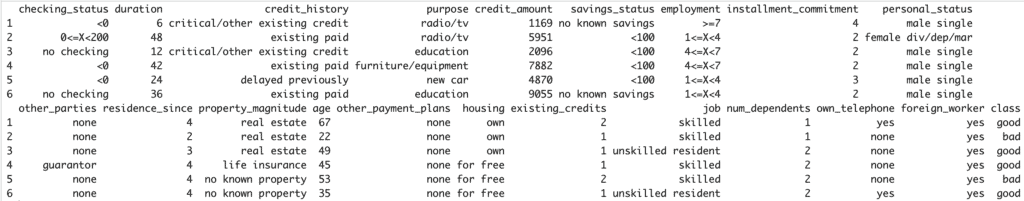### Step 2: Plotting a Simple Strip Chart

After analyzing the data, we will create a strip chart for the credit_amount by credit_history.

``````# Load the dataset

# Strip chart using stripchart() function
stripchart(credit_amount ~ credit_history,
data = credit_data,
pch = 16,
col = 'blue',
main = "Credit Amount by Credit History",
xlab = "Credit Amount",
ylab = "Credit History")``````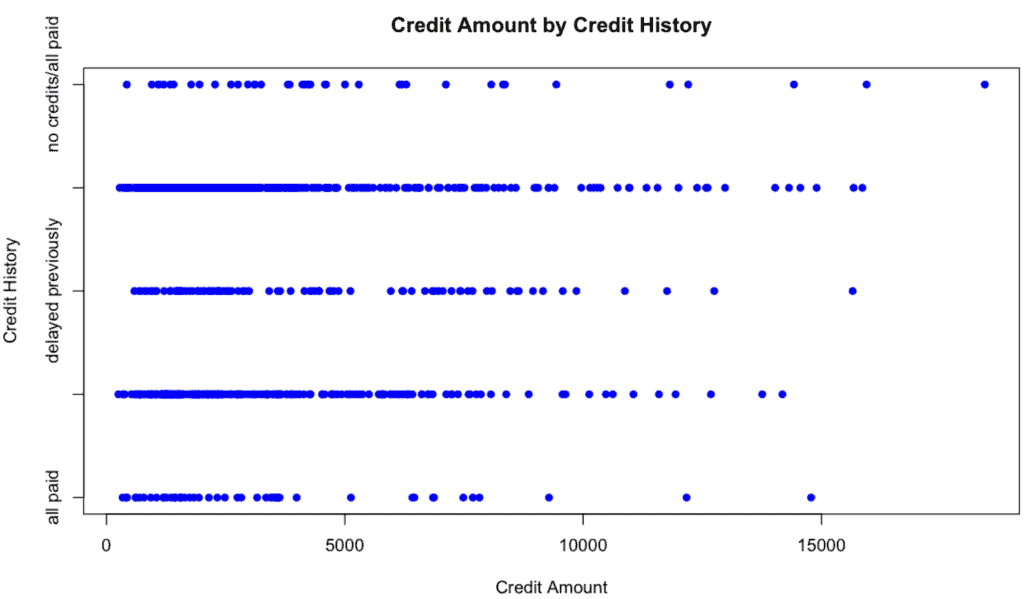Explanation:

1. pch = 16: This sets the plotting character to a solid circle.
2. col = “blue”: This sets the color of the points to blue.

## Example 2: Create a vertical strip chart

To create a vertical strip chart using the stripchart() function, you can use the vertical = TRUE argument.

``````# Vertical Strip chart using stripchart() function
stripchart(credit_amount ~ credit_history, data = credit_data,
vertical = TRUE,
pch = 16,
col = 'blue',
main = "Credit Amount by Credit History",
xlab = "Credit Amount",
ylab = "Credit History")``````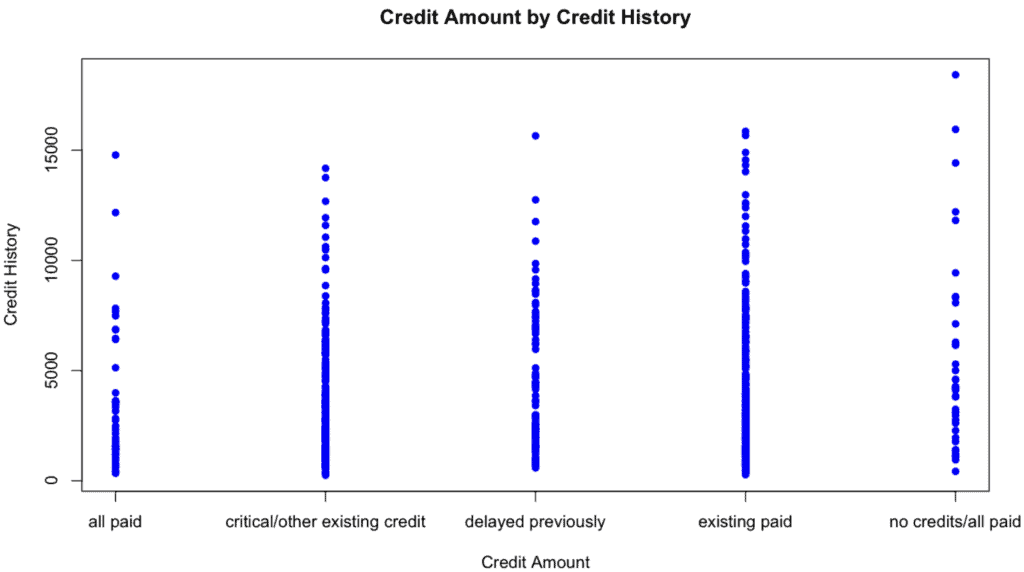## Example 3: Create a strip chart without overlapping points with the method argument set to stack

``````# Vertical Strip chart using stripchart() function with method set to stack
stripchart(credit_amount ~ credit_history, data = credit_data,
vertical = TRUE,
method = "stack",
pch = 16,
col = 'blue',
main = "Credit Amount by Credit History",
xlab = "Credit Amount",
ylab = "Credit History")``````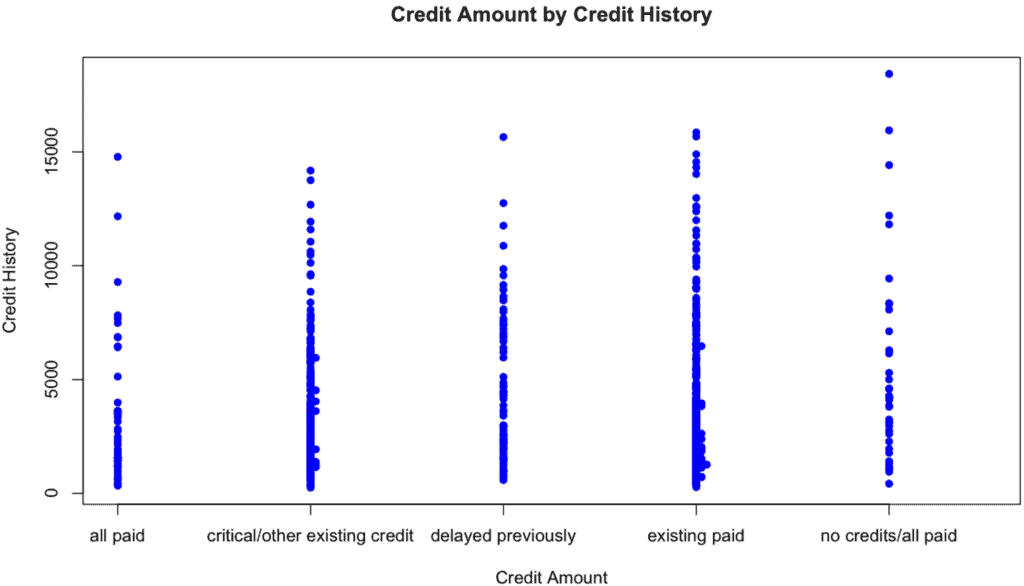In this code, method = “stack” is used to stack points vertically with the same value.

## Example 4: Strip chart using jitter

The jitter argument in the stripchart() function introduces some randomness to the position of the points to reduce overlap. This is especially helpful when you have a lot of data points with the same or very close values.

``````# Load the dataset

# Vertical Strip chart using stripchart() with jitter
stripchart(credit_amount ~ credit_history, data = credit_data,
vertical = TRUE,
method = "jitter",
pch = 16,
col = 'blue',
main = "Credit Amount by Credit History",
xlab = "Credit Amount",
ylab = "Credit History")``````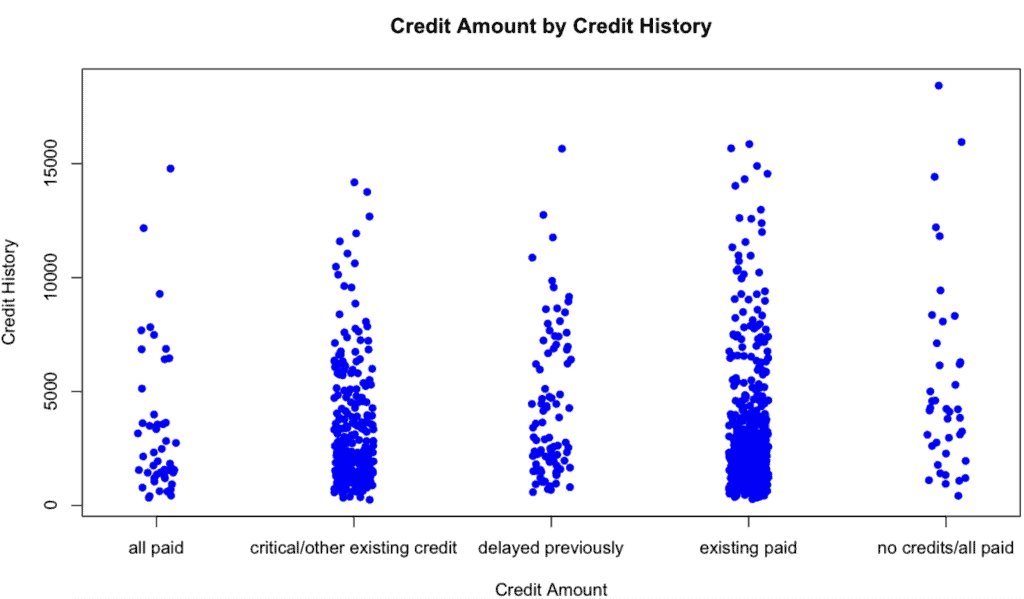In this code method = “jitter” introduces randomness to the position of the points to reduce overlap.

## Example 5: Multiple Strip Charts

To create multiple strip charts for different groups, you can use the stripchart() function in combination with the par() function, which sets or queries graphical parameters.

For example, if you would like to create multiple strip charts for credit_amount grouped by credit_history and further differentiated by job, you can do the following:

``````# Load the dataset

# Set up the graphics window to display 2x2 plots
par(mfrow = c(2, 2))

# List of unique job types
job_types <- unique(credit_data\$job)

# Loop through job types and create a strip chart for each
for (job in job_types) {
subset_data <- credit_data[credit_data\$job == job, ]
stripchart(credit_amount ~ credit_history, data = subset_data,
vertical = TRUE,
method = "jitter",
pch = 16,
col = 'blue',
main = paste("Credit Amount by Credit History (Job:", job, ")"),
xlab = "Credit Amount",
ylab = "Credit History")
}``````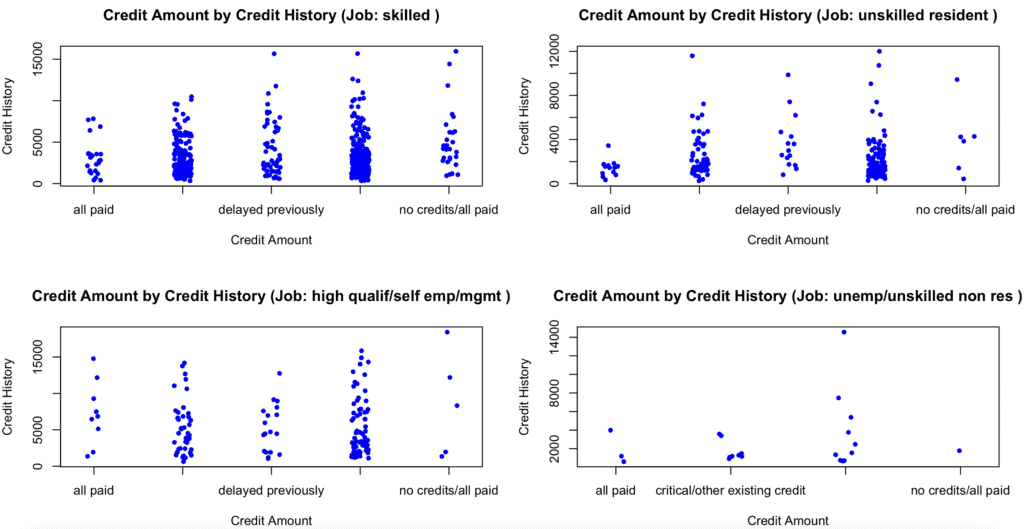You can see from the above chart that we divided the graphics window into a 2×2 grid (or other dimensions depending on the number of unique job types) and displayed a strip chart for each.

You can analyze various things like which job is getting good credit and which is getting no credit.

## Conclusion

A strip chart is a one-dimensional scatter plot, ideal for plotting data that isn’t too dense. It helps visualize the distribution of data points along a single axis, often categorized by some factor.